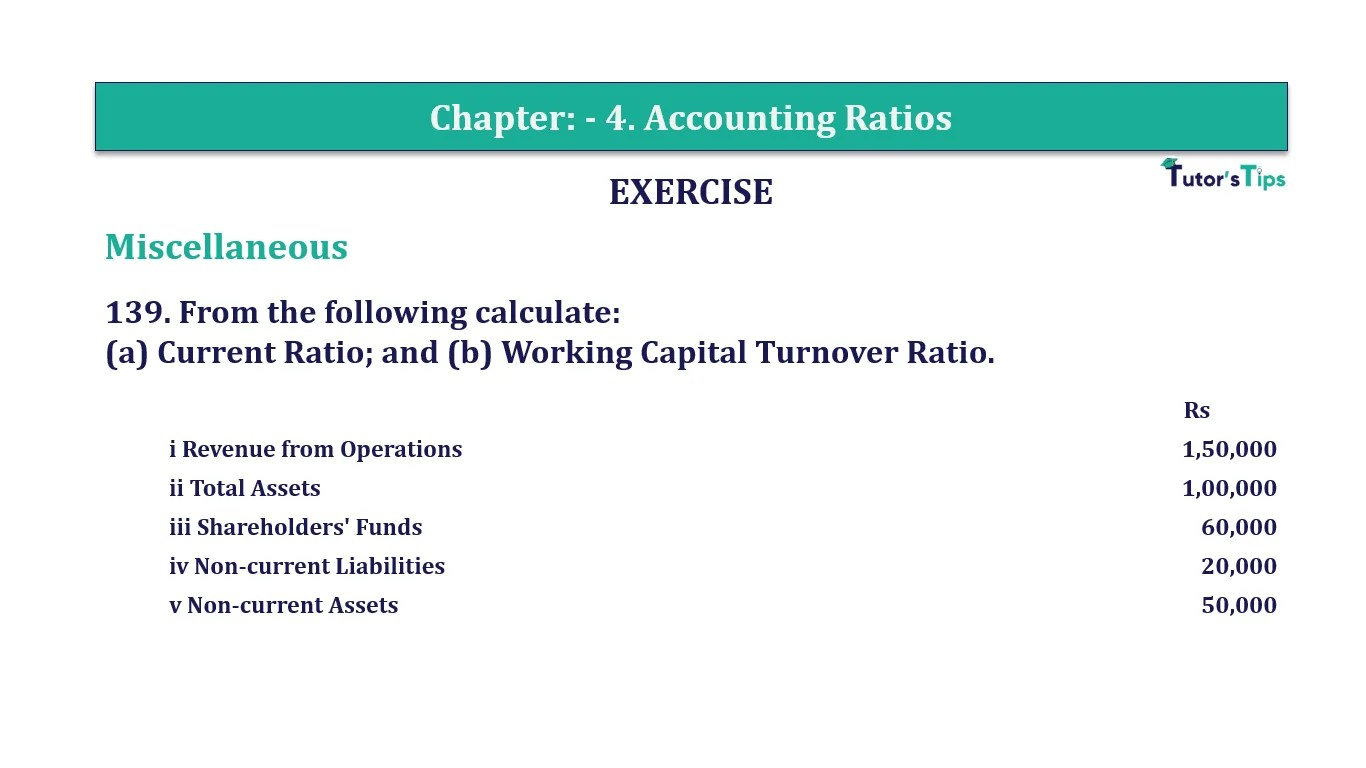# Question 139 Chapter 4 of +2-B – T.S. Grewal 12 ClassQuestion No. 139- Chapter No.4 - T.S. Grewal +2 Book Part B

Question 139 Chapter 4 of +2-B

Miscellaneous

139. From the following calculate:
(a) Current Ratio; and (b) Working Capital Turnover Ratio.

 Rs i Revenue from Operations 1,50,000 ii Total Assets 1,00,000 iii Shareholders’ Funds 60,000 iv Non-current Liabilities 20,000 v Non-current Assets 50,000

### The solution of Question 139 Chapter 4 of +2-B: –

 Current Assets = Total Assets – Non Current Assets = Rs. 1,00,000 – Rs 50,000 = Rs 50,000 Total Liabilities = Shareholders’ Funds + Non Current Liabilities + Current Liabilities Rs 1,00,000 = Rs. 60,000 + Rs 20,000 + Current Liabilities Current Liabilities = Rs 1,00,000 – Rs 80,000 = Rs 20,000

 Current Ratio = Current Assets Current Liabilities
 Current Ratio = Rs. 50,000 Rs 20,000 = 2.5 : 1

 Working Capital = Current Assets – Current Liabilities = Rs 50,000 − Rs 20,000 = Rs 30,000
 Working Capital Turnover Ratio = Revenue from Operations Working Capital
 = Rs 1,50,000 Rs 30,000 = 5 Times

Balance Sheet: Meaning, Format & Examples

Comment if you have any question.

Also, Check out the solved question of previous Chapters: –